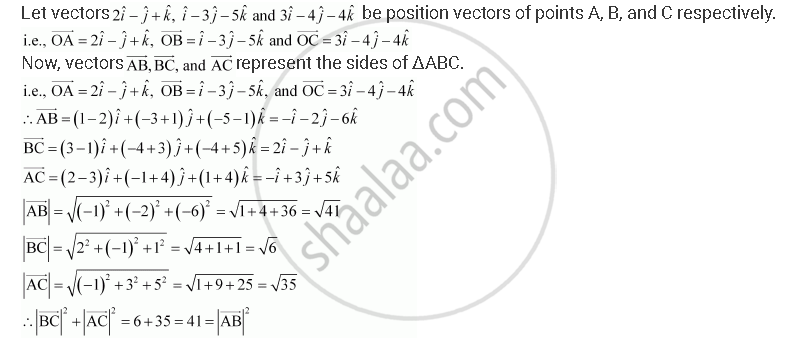Share

# Show that the Vectors 2hayti - Hatj + Hatk and 3hati - 4hatj - 4hatk Form the Vertices of a Right Angled Triangle. - CBSE (Commerce) Class 12 - Mathematics

ConceptIntroduction of Product of Two Vectors

#### Question

Show that the vectors 2hati - hatj + hatk and 3hati - 4hatj - 4hatk form the vertices of a right-angled triangle.

#### SolutionHence, ΔABC is a right-angled triangle.

Is there an error in this question or solution?

#### Video TutorialsVIEW ALL 

Solution Show that the Vectors 2hayti - Hatj + Hatk and 3hati - 4hatj - 4hatk `Form the Vertices of a Right Angled Triangle. Concept: Introduction of Product of Two Vectors.
S